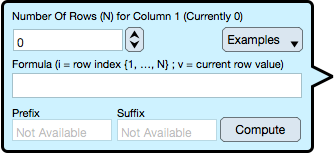Graph IDEControls ► Formula Selector

The Formula Selector computes a sequence of values for a Spreadsheet and Tables column. It is important to note that a formula is a quick way to populate a column of values and that once populated then each table cell entry can be further modified.

For making more general algorithms see Programming.

Formula Selector

The Formula Selector is brought forward by selecting the "Formula" entry to the Component drop down. The Component drop down is brought forward by right-mouse-click or select-hold on a table column header or by using the Component drop down menu near the table. The Formula Selector is annotated below.Number Of Rows : The number of times that the formula is computed. Upon each execution the domain index ('i' which represents the row index of a column in a table) is incremented by one.

Examples : Gives a menu of possible formula entries. Select a menu item to load that entry. The last entry in the menu is "Revert to formatter settings" and if selected loads the formula last stored in a Spreadsheet column.

Formula : A formula that uses the symbol 'i' as its domain and utilizes the Programming facilities to compute new range values. The current range of the row is represented by the symbol 'v'. A formula is a single statement program.

Prefix : The prefix to the range of the formula. If the table column is numeric only type then the prefix is not available.

Suffix : The suffix to the range of the formula. If the table column is numeric only type then the suffix is not available.

Compute : Once all parameters have been entered then select the Compute button to perform the formula mapping and enter all values into the selected table column.

Formula Examples

Example formulas are shown in the table below. For language syntax and predefined function declarations see Language.

 Formula Description i Fill with row index 1 to 'N'. Notice that the domain variable begins at 1 and increments by 1 to the value of 'N' -v Reverse the sign of the current values. This is short for -1 * v. 2 * v Double current values. Any algebraic formula can be used so that the formula can be quite complex. Use the normal parenthesis to define precedence of operation. floor(v * 100 + 0.5)/100 Round current values to two decimal places. floor() means make its argument a whole number by truncation. The function ceil() means make its argument a whole number by increasing to the next whole number. To be explicit, making a whole number from a real number has two operators instead of the normal one. i % 2 Alternate between 1 and 0. The percentage symbol is the modulus operator. 1 Fill with the constant 1. Any constant number can be used. sin((i - 1.0) * 0.1) Fill with a modulated signal. The Language section list all available functions. NAN Invalidates all rows. Note that NAN must be all capitals to be interpreted as the "Not a Number" entry. This is in opposition to entering NaN into a table cell as that is case insensitive.

Note that the symbol 'v' is the current value of the table row and must be a numeric value in order for the formula to be valid. If the table column is not of a numeric type then 'v' can not be used.

Here are a few ideas:

• If the Prefix is set to the word "Sample " (with a trailing space but with no quotes) and then 'i' (without the single quotes) is used as the formula then the column entries with be computed as "Sample 1", "Sample 2" ... "Sample N" which is appropriate for entries to a Legend for a graph.

• If 'i % 2' (without the single quotes) is used then the value alternates between 1 and 0 which may be appropriate for assigning values to the rgba components of segments of a Function. The constant '1' (without the single quotes) can be used to assign a solid alpha component to the color.

• Sometimes data is pasted into a Data Graphic and the coordinate is flipped, particularly the y-value coordinate. Applying '-v' (without the single quotes) flips the data values associated with that coordinate and table column. Sometimes data has two components (x and y) and both are sampled irregularly but it may be desirable to make one component regular (have regular intervals). Applying something like 'i', '100 * i' or some variation of that will make that coordinate values regular (uniform, of constant increment, et.al.).

• Formulas are very powerful, useful and specific. For more general programming see Programming. In particular, a program is a multi-statement algorithm (a sequence of functions) which can be animated (has an additional index symbol associated with animation step a.k.a. time). When programs are combined with loading a Plugin (a shared object library) then data can be automated, fetched and animated from a variety of sources and not just an algorithm.